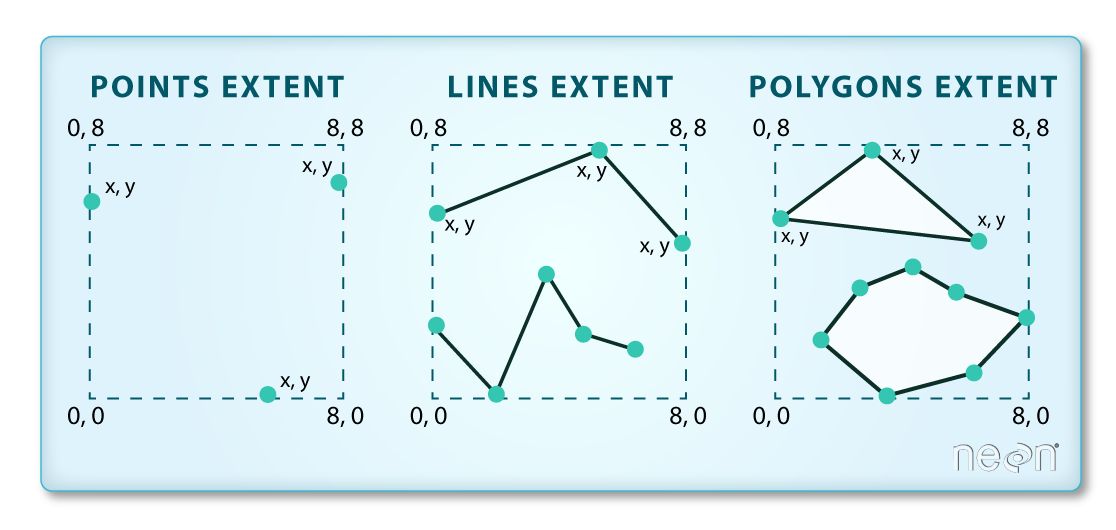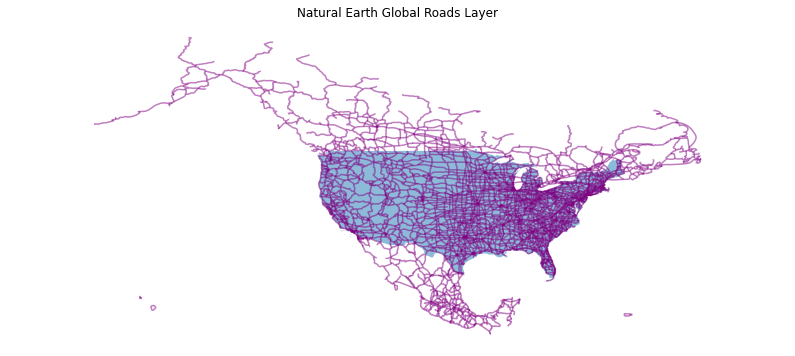# Lesson 2. Customize your Maps in Python: GIS in Python

## Learning Objectives

After completing this tutorial, you will be able to:

• Clip a spatial vector point and line layer to the spatial extent of a polygon layer in Python
• Visually “clip” or zoom in to a particular spatial extent in a plot.

## What You Need

You will need a computer with internet access to complete this lesson and the spatial-vector-lidar data subset created for the course.

or using the earthpy package: et.data.get_data("spatial-vector-lidar")

In this lesson, you will learn how to spatially clip data for easier plotting and analysis of smaller spatial areas. You will use the geopandas library and the box module from the shapely library.

# Import libraries
import os
import numpy as np
import matplotlib.pyplot as plt
import geopandas as gpd
import earthpy as et

# Get the data
data = et.data.get_data('spatial-vector-lidar')

os.chdir(os.path.join(et.io.HOME, 'earth-analytics', 'data'))

Downloading from https://ndownloader.figshare.com/files/12459464
Extracted output to /root/earth-analytics/data/spatial-vector-lidar/.

# Read in necessary files
"california",
"neon-sjer-site",
"vector_data",
"SJER_crop.shp"))
"usa",
"usa-boundary-dissolved.shp"))
"global",


## Change the Spatial Extent of a Plot in Python

Above you modified your data by clipping it. This is useful when you want to

1. Make your data smaller to speed up processing and reduce file size
2. Make analysis simpler and faster given less data to work with.

However, if you just want to plot the data, you can consider adjusting the spatial extent of a plot to “zoom in”. Note that zooming in on a plot does not change your data in any way - it just changes how your plot renders!

To zoom in on a region of your plot, you first need to grab the spatial extent of the object

# Get spatial extent  - to zoom in on the map rather than clipping
aoi_bounds = sjer_aoi.geometry.total_bounds
aoi_bounds

array([ 254570.567     , 4107303.07684455,  258867.40933092,
4112361.92026107])


The total_bounds attribute represents the total spatial extent for the aoi layer. This is the total external boundary of the layer - thus if there are multiple polygons in the layer it will take the furtherst edge in the north, south, east and west directions to create the spatial extent box.The spatial extent of a shapefile or Python spatial object like a geopandas geodataframe represents the geographic "edge" or location that is the furthest north, south east and west. Thus is represents the overall geographic coverage of the spatial object. Image Source: National Ecological Observatory Network (NEON)

The object that is returned is a tuple - a non editable object containing 4 values: (xmin, ymin, xmax, ymax). If you want you can assign each value to a new variable as follows

# Create x and y min and max objects to use in the plot boundaries
xmin, ymin, xmax, ymax = aoi_bounds

fig, ax = plt.subplots(figsize  = (14, 6))
country_boundary_us.plot(alpha = .5, ax = ax)
ax.set(title='Natural Earth Global Roads Layer')
ax.set_axis_off()
plt.axis('equal')
plt.show()Plot of major North American roads in Canada, the United States, and Mexico, plotted on top of the boundary of the United States.

You can set the x and ylimits of the plot using the x and y min and max values from your bounds object that you created above to zoom in your map.

# Use the country boundary to set the min and max values for the plot
country_boundary_us.total_bounds

array([-124.725839,   24.498131,  -66.949895,   49.384358])


Notice in the plot below, you can still see roads that fall outside of the US Boundary area but are within the rectangular spatial extent of the boundary layer. Hopefully this helps you better understand the difference between clipping the data to a polygon shape vs simply plotting a small geographic region.

# Plot the data with a modified spatial extent
fig, ax = plt.subplots(figsize = (10,6))
xlim = ([country_boundary_us.total_bounds,  country_boundary_us.total_bounds])
ylim = ([country_boundary_us.total_bounds,  country_boundary_us.total_bounds])

ax.set_xlim(xlim)
ax.set_ylim(ylim)

country_boundary_us.plot(alpha = .5, ax = ax)